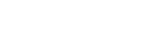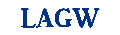# STATA ASSIGNMENT HELP

STATA

STATA is a comprehensive statistical package that provides everything you may need about data analysis, graphics, data management, custom programming and simulation. STATA assignment help provides solution to the following advanced techniques: survival models, generalized estimating equations, multiple imputation, models with sample selection, dynamic panel data regression, multilevel mixed models, estimation with complex survey samples, and ARCH assignment. STATA homework help under the standard methods include: linear and generalized linear models, ANOVA/MANOVA, standardization of rates, basic tabulation and summary statistics, cluster analysis, and regression with count ad binary outcomes.

Help in STATA ranges from the basic which includes functions, and language syntax; statistics includes: tables, summary equation and estimation; graphics includes bar charts and scatterplots; data management from inputting, creating new variables and editing; and programming and matrices from mata, matrices, do and ado files. STATA help regression analysis involves following specific steps which are the following: first, is obtaining some data. Second is converting the obtained data into STATA data set. Next is to run the regression and plotting the prediction and lastly is to examine some of the useful regression diagnostics. STATA graph help can be obtained through using specific commands that could display the graph itself after the inputs are made.

### STATA Assignment Help

Statisticshelpdesk offers online STATA assignment help in all topics related to statistics. Whether its basics, analysis, regression our tutors make students grasp the concepts and understand the application thoroughly. Our STATA Assignment help services comprises of all solution to complex problems associated with STATA. Our step by step approach helps students to understand the solution themselves. We provide STATA Assignment help through email where a student can quickly upload his STATA Homework on our website and get it done before the due date.

### STATA Homework Help

STATA Homework Help covers all homework and course work questions in STATA. Our tutors are highly efficient in teaching the use and application of STATA software on robust online platform. Students can learn to get the best advantage out of STATA software in solving various statistical problems. Our online STATA Homework help is a one stop solution to get last minute help in exams, practicals, quizzes and tests.

### STATA Online Tutor

Our STATA online tutors are highly experienced statistics tutors with over three years of academic teaching experience as well as research. We provide STATA Tutor Help online service in which a student can have a direct interaction with our tutors online in the form of live chatting and online session. The student can take the advantage of exam preparation and seek help in his/her quizzes and tests. We provide convenient and easy services at affordable session rates to students seeking help from online Statistics tutor.

SUBMIT HOMEWORK
• Submit your homework for a free quote
• Name:*
• Email:*
• Phone:*
• Subject:*Change Code